# The Capital Asset Pricing Model Revisited¶

## Background Info¶

What follows is based on the Quantopian Lecture 30 on the Capital Asset Pricing Model by Beha Abasi, Maxwell Margenot, and Delaney Granizo-Mackenzie. Thanks to all for the notebook.

The above notebook gives a graphic depiction of a Markowitz bullet and illustrates the concept behind the portfolio efficient frontier. I have nothing against that. On the contrary, it's the classic representation of the expectations or the set of forward objectives one should consider when determining the possible optimal allocations on a small group of stocks in a portfolio.

Markowitz BulletEach dot in the above chart is one of the 50,000 portfolios represented. All were simulated, each based on 4 stocks having randomly distributed price series.

In the original notebook, all return streams were randomly generated based on a normal (Gaussian) distribution. There is nothing wrong with that. It simply normalized all price series to behave comparatively on the same basis since the interest is only on the percentage change from period to period of the underlying securities.

I have not changed any of the main code. Only some of the controlling parameters, namely: the number of assets (stocks) to be used, the number of observations made, and the number of simulated portfolios.

## Simulation Environment¶

Looking at 4 stocks is not that realistic a scenario in an environment looking to create portfolios having in excess of 100 stocks. Going to even more than a thousand stocks has recently been suggested as desirable by Quantopian.

There was no need to simulate 50,000 portfolios, so I reduced it to 5,000. It should still be more than enough to make the point. Also reduced the number of observations from 2,000 to 1,000. Just trying to save some processing time on that one. Doing a 1,000-stock test can take quite a while.

The changed numbers control the number of iterations and not the structure of the program itself. So, there is no loss of generalities with the changes I've made.

The main change, however, and having a real impact, is the increase to 100 stocks per simulated portfolio.

Just as in the original notebook, the classic CAPM equation holds:

$$E[R_i] = R_F + \beta(E[R_M] - R_F)$$

And, as was said:

CAPM says that the return of an asset should be the risk-free rate, which is what we would demand to account for inflation and the time value of money, as well as something extra to compensate us for the amount of systematic risk we are exposed to.

The same CAPM assumptions as in the original notebook are maintained since I have not changed them either.

We assumed that investors are able to trade without delay or cost and that everyone is able to borrow or lend money at the risk-free rate.

We assumed that all investors are "mean-variance optimizers". What this essentially means is that they would only demand portfolios that have the highest return attainable for a given level of risk. These portfolios are all found along the efficient frontier.

The following is a programmatic derivation of the efficient frontier for portfolios of four assets.

See the CAPM notebook for more detail.

## Scenario Analysis¶

In :
# Import libraries
import numpy as np
import pandas as pd
import statsmodels.api as sm
from statsmodels import regression
import matplotlib.pyplot as plt

from scipy import optimize
import cvxopt as opt
from cvxopt import blas, solvers


The original scenario has been changed to account for 10 and then 100 stocks instead of just 4 as above. The number of iterations was reduced to 1,000. The number of portfolios to be generated was reduced to 5,000. And $\mathsf{N}$ was also reduced to 1,000. For everything else, the program is the same.

Let's look at the scenario where only 10 stocks are considered in order to gain a better understanding of the general behavior and transition from 4 to 10 (below) and then to 100 stocks.

In :
np.random.seed(123)

# Turn off progress printing
solvers.options['show_progress'] = False

# Number of assets
n_assets = 10

# Number of observations
n_obs = 1000

## Generating random returns for the securities
return_vec = np.random.randn(n_assets, n_obs)

def rand_weights(n):
'''
Produces n random weights that sum to 1
'''
k = np.random.rand(n)
return k / sum(k)

def random_portfolio(returns):
'''
Returns the mean and standard deviation of returns for a random portfolio
'''

p = np.asmatrix(np.mean(returns, axis=1))
w = np.asmatrix(rand_weights(returns.shape))
C = np.asmatrix(np.cov(returns))

mu = w * p.T
sigma = np.sqrt(w * C * w.T)

# This recursion reduces outliers to keep plots pretty
if sigma > 2.0:
return random_portfolio(returns)
return mu, sigma

def optimal_portfolios(returns):
n = len(returns)
returns = np.asmatrix(returns)

N = 1000

# Creating a list of returns to optimize the risk for
mus = [100**(5.0 * t/N - 1.0) for t in range(N)]

# Convert to cvxopt matrices
S = opt.matrix(np.cov(returns))
pbar = opt.matrix(np.mean(returns, axis=1))

# Create constraint matrices
G = -opt.matrix(np.eye(n))   # negative n x n identity matrix
h = opt.matrix(0.0, (n ,1))
A = opt.matrix(1.0, (1, n))
b = opt.matrix(1.0)

# Calculate efficient frontier weights using quadratic programming
portfolios = [solvers.qp(mu*S, -pbar, G, h, A, b)['x']
for mu in mus]

## Calculate the risk and returns of the frontier
returns = [blas.dot(pbar, x) for x in portfolios]
risks = [np.sqrt(blas.dot(x, S*x)) for x in portfolios]

return returns, risks

n_portfolios = 5000

means, stds = np.column_stack([random_portfolio(return_vec) for x in range(n_portfolios)])

returns, risks = optimal_portfolios(return_vec)

plt.plot(stds, means, 'o', markersize=2, color='navy')
plt.xlabel('Risk')
plt.ylabel('Return')
plt.title('Mean and Standard Deviation of Returns of Randomly Generated Portfolios');

plt.plot(risks, returns, '-', markersize=3, color='red');
plt.legend(['Portfolios', 'Efficient Frontier']);

# Markowitz Bullet:  10 Stocks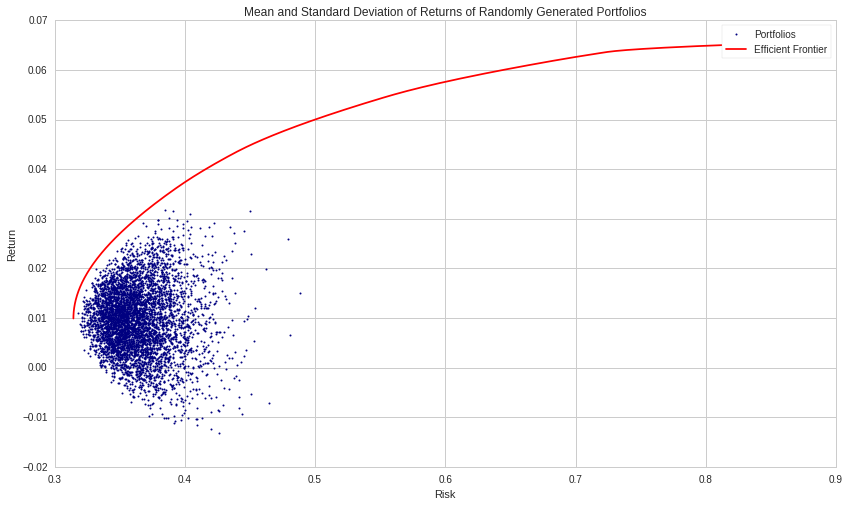The above chart still shows the Markowitz bullet. But, it has been considerably compacted.

It is even more so for the 100-stock case as illustrated below.

In :
np.random.seed(123)

# Turn off progress printing
solvers.options['show_progress'] = False

# Number of assets
n_assets = 100

# Number of observations
n_obs = 1000

## Generating random returns for the securities
return_vec = np.random.randn(n_assets, n_obs)

def rand_weights(n):
'''
Produces n random weights that sum to 1
'''
k = np.random.rand(n)
return k / sum(k)

def random_portfolio(returns):
'''
Returns the mean and standard deviation of returns for a random portfolio
'''

p = np.asmatrix(np.mean(returns, axis=1))
w = np.asmatrix(rand_weights(returns.shape))
C = np.asmatrix(np.cov(returns))

mu = w * p.T
sigma = np.sqrt(w * C * w.T)

# This recursion reduces outliers to keep plots pretty
if sigma > 2.0:
return random_portfolio(returns)
return mu, sigma

def optimal_portfolios(returns):
n = len(returns)
returns = np.asmatrix(returns)

N = 1000

# Creating a list of returns to optimize the risk for
mus = [100**(5.0 * t/N - 1.0) for t in range(N)]

# Convert to cvxopt matrices
S = opt.matrix(np.cov(returns))
pbar = opt.matrix(np.mean(returns, axis=1))

# Create constraint matrices
G = -opt.matrix(np.eye(n))   # negative n x n identity matrix
h = opt.matrix(0.0, (n ,1))
A = opt.matrix(1.0, (1, n))
b = opt.matrix(1.0)

# Calculate efficient frontier weights using quadratic programming
portfolios = [solvers.qp(mu*S, -pbar, G, h, A, b)['x']
for mu in mus]

## Calculate the risk and returns of the frontier
returns = [blas.dot(pbar, x) for x in portfolios]
risks = [np.sqrt(blas.dot(x, S*x)) for x in portfolios]

return returns, risks

n_portfolios = 5000

means, stds = np.column_stack([random_portfolio(return_vec) for x in range(n_portfolios)])

returns, risks = optimal_portfolios(return_vec)

plt.plot(stds, means, 'o', markersize=2, color='navy')
plt.xlabel('Risk')
plt.ylabel('Return')
plt.title('Mean and Standard Deviation of Returns of Randomly Generated Portfolios');

plt.plot(risks, returns, '-', markersize=3, color='red');
plt.legend(['Portfolios', 'Efficient Frontier']);

# Markowitz Bullet:  100 Stocks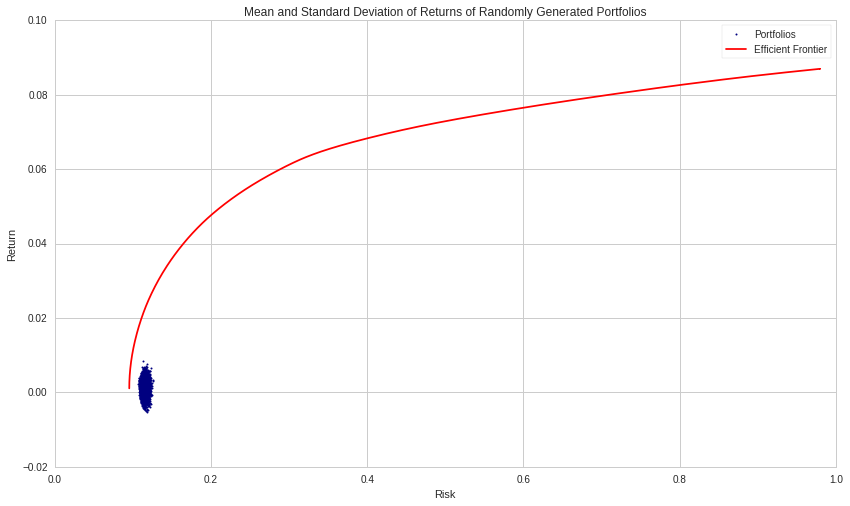The above chart should be compared to the first one above where you have a more full-blown Markowitz bullet which was referenced in the CAPM notebook.

The following is from the original notebook.

Each blue dot represents a different portfolio, while the red line skimming the outside of the cloud is the efficient frontier. The efficient frontier contains all portfolios that are the best for a given level of risk.

The optimal, or most efficient, portfolio on this line is found by maximizing the Sharpe ratio, the ratio of excess return and volatility. We use this to determine the portfolio with the best risk-to-reward tradeoff.

The line that represents the different combinations of a risk-free asset with a portfolio of risky assets is known as the Capital Allocations Line (CAL). The slope of the CAL is the Sharpe ratio. To maximize the Sharpe ratio, we need to find the steepest CAL, which coincides with the CAL that is tangential to the efficient frontier. This is why the efficient portfolio is sometimes referred to as the tangent portfolio.

The last chart above cries for a different interpretation.

By increasing the number of stocks to be considered, we see the original Markowitz bullet slowly shrinking and migrating to the above chart location after having reached 100 stocks. It will stay as compact, get even tighter when adding more stocks. The density will, however, continue to increase.

Zooming in on the above chart, we can still see a Markowitz bullet, but now, its mass is centered around zero. Almost saying: you are taking some risk (albeit small) with an expected return somewhere near zero (in all expectations). It holds for the 5,000 randomly generated portfolios. It is a sufficiently large number to give it statistical significance.

This is quite in contrast to the original notebook (see the first chart above). Regardless, what is shown in the last chart is not what the theory says.

The optimizer did its job when looking at 4 securities, it did its job at 10, and again at 100. Nonetheless, it is not what you would have expected. You wanted some of those dots to hog the efficient frontier and have a bit more of a profit opportunity than zero.

Optimizing on 100 stocks simply obliterated performance.

Therefore, it does raise a big question. What does it all mean?

If you plot a single return stream, we can observe its random signature. Most of it within 2 standard deviations (as should be expected) since the series were clipped at that level in order to minimize the impact of outliers. Overall, it did not make much of a difference.

In :
# Plot a single return vector (normally distributed).
# Pick a number between 1 and (n_assets -1).
picked_ret_vec = 42
plt.plot(return_vec[picked_ret_vec]);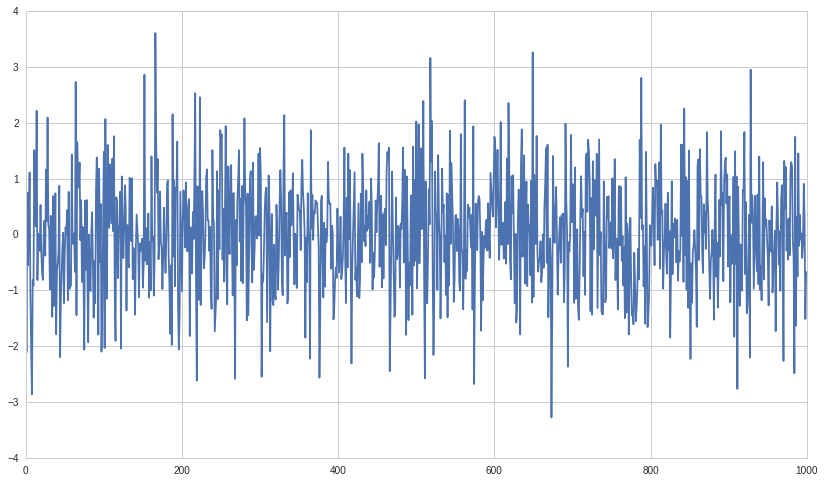In :
#Plot selected return stream as if an index, since only the percentages are of interest.
plt.plot(np.cumsum(return_vec[picked_ret_vec])+100);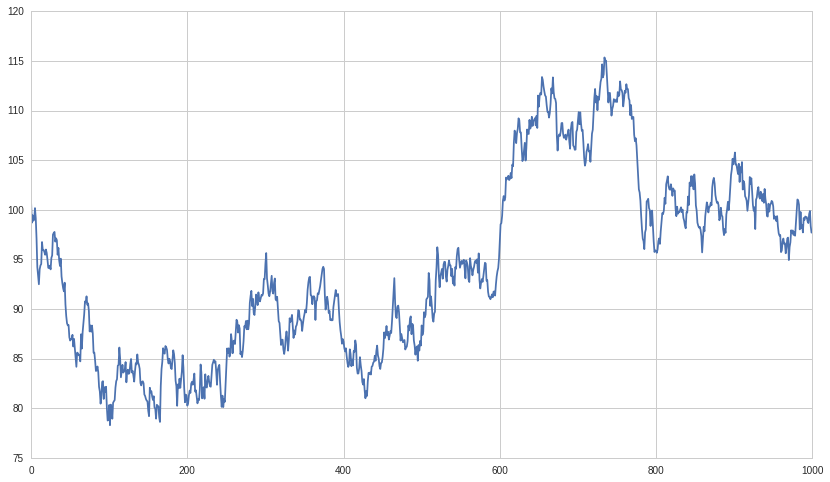## Maximizing the Sharpe Ratio¶

Maximizing the Sharpe Ratio might be an objective, but is it really, under such a scenario?

In :
# first get a risk-free rate of return proxy
start_date = '2014-01-01'
end_date = '2016-12-31'

R_F = get_pricing('BIL', fields='price', start_date=start_date, end_date=end_date).pct_change()[1:]


Nothing was changed in the next section of code. It does use the same return_vec, and therefore it should complement the previous Markowitz bullet chart.

In :
def maximize_sharpe_ratio(return_vec, risk_free_rate):
"""
Finds the CAPM optimal portfolio from the efficient frontier
by optimizing the Sharpe ratio.
"""

def find_sharpe(weights):

means = [np.mean(asset) for asset in return_vec]

numerator = sum(weights[m]*means[m] for m in range(len(means))) - risk_free_rate

weight = np.array(weights)

denominator = np.sqrt(weights.T.dot(np.corrcoef(return_vec).dot(weights)))

return numerator/denominator

guess = np.ones(len(return_vec)) / len(return_vec)

def objective(weights):
return -find_sharpe(weights)

# Set up equality constrained
cons = {'type':'eq', 'fun': lambda x: np.sum(np.abs(x)) - 1}

# Set up bounds for individual weights
bnds = [(0, 1)] * len(return_vec)

results = optimize.minimize(objective, guess,
constraints=cons, bounds=bnds,
method='SLSQP', options={'disp': False})

return results

risk_free_rate = np.mean(R_F)

results = maximize_sharpe_ratio(return_vec, risk_free_rate)

# Applying the optimal weights to each assset to get build portfolio
optimal_mean = sum(results.x[i]*np.mean(return_vec[i]) for i in range(len(results.x)))

optimal_std = np.sqrt(results.x.T.dot(np.corrcoef(return_vec).dot(results.x)))

# Plot of all possible portfolios
plt.plot(stds, means, 'o', markersize=2, color='navy')
plt.ylabel('Return')
plt.xlabel('Risk')

# Line from the risk-free rate to the optimal portfolio
eqn_of_the_line = lambda x : ( (optimal_mean-risk_free_rate) / optimal_std ) * x + risk_free_rate

xrange = np.linspace(0., 1., num=11)

plt.plot(xrange, [eqn_of_the_line(x) for x in xrange], color='red', linestyle='-', linewidth=2)

# Our optimal portfolio
plt.plot([optimal_std], [optimal_mean], marker='o', markersize=12, color="navy")

plt.legend(['Portfolios', 'Capital Allocation Line', 'Optimal Portfolio']);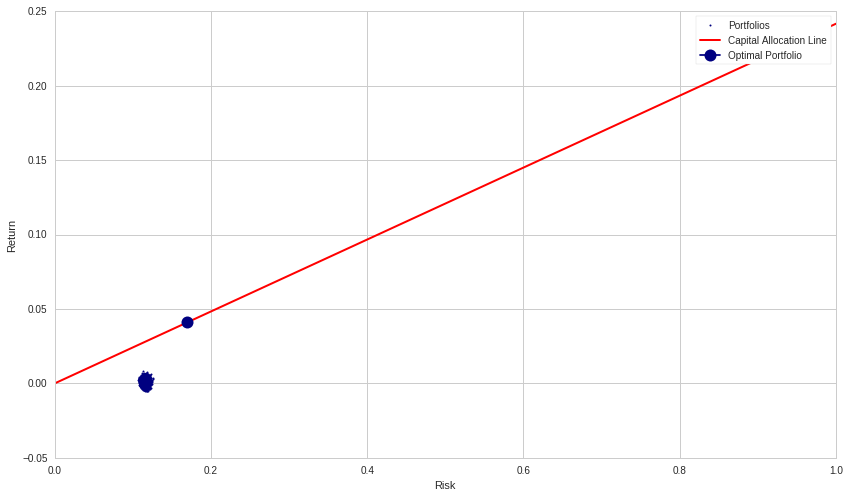The above chart is consistent with the generated data. There might have been an optimal portfolio, but it was not reachable. All you have is this small blob of 5,000 data points centered around the zero-return line.

The original notebook said:

We can look at the returns and risk of the individual assets compared to the optimal portfolio we found to easily showcase the power of diversification.

The power of diversification, even if technically it is still there, did not show that much power. In fact, practically none at all.

It is as if saying that whatever portfolio you took out of the 5,000 considered, the expected outcome would be about the same or at least very close to all the others. And therefore, why not simply make a bet, whatever it is. In the end, it will not matter much. Especially since you do not have any means to figure out which will turn out to be the best of the group anyway. Even if you did, it would be for peanuts, literally peanuts.

In :
# show the first 10 assets of the return vector
for a in range(len(return_vec[:10])):
print "Return and Risk of Asset ", a, ":", np.mean(return_vec[a]), \
",",np.std(return_vec[a])

print "\nReturn and Risk of Optimal Portfolio", optimal_mean, optimal_std

# the standard deviation should be close to 1.00 by design.

Return and Risk of Asset  0 : -0.0395641360808 , 1.00078753752
Return and Risk of Asset  1 : 0.00838916739587 , 0.958009555142
Return and Risk of Asset  2 : 0.010353090377 , 0.981458372151
Return and Risk of Asset  3 : 0.0664786870526 , 0.989044689799
Return and Risk of Asset  4 : 0.0597576253925 , 1.00502947026
Return and Risk of Asset  5 : -0.018464149794 , 0.977131008917
Return and Risk of Asset  6 : 0.0290177194568 , 0.996084354406
Return and Risk of Asset  7 : -0.0378914504098 , 1.00899739047
Return and Risk of Asset  8 : 0.0346915023353 , 1.04790835915
Return and Risk of Asset  9 : -0.0156491328094 , 1.00766393381

Return and Risk of Optimal Portfolio 0.0409602848882 0.169471481335


## Some Explanation Required¶

A stochastic price equation could be written as: $p(t) = \mu dt + \sigma dW$. Again, a classic, which is a simple regression line to which is added some random stuff (noise). However, if you remove $\mu dt$ (detrending the price series) as in the above scenario, you are left with $p(t) = \sigma dW$, the random component of the stochastic price function. And there, the overall expectancy is zero.

That you take 4 such series, a 100, or even a thousand, the expected outcome will still be the same, that is zero. That you take them individually or collectively, it will not change the expected outcome. Even if you increased the number of observations to 2,000 you would get the same picture.

From normally generated random price series, there is nothing to be had. And I think the above showed exactly that. It would also suggest that eliminating the notion of secular trends in the stochastic price function is not conducive to provide any serious alpha from a return optimizer. Meaning that if you want to treat price series as random-walks, don't expect more than a zero expectancy.

This is an example of when looking small, you do not see the big picture. Should this have surprised anyone? Actually, no. From the actual premises, it should have been expected. I was surprised that the original notebook could picture a nice and smooth Markowitz bullet in the first place.

OR, should I understand all this as some kind of error in the code?

Presently, I see it more as the "expected" outcome of the optimizer. You want to flatten out the variance of randomly generated price series with no underly secular trends in order to diversify your portfolio, then the above is what will happen.

The optimizer followed all the constraints, even tried to outguess what was coming, but to no avail. How could it? Returns were randomly generated with no predictability available. So, the optimizer did its best and optimized what was available, and that is nothing.

For the complete Jupyter notebook where you can modify the program code, clone the attached notebookin the Quantopian thread. (Registration on Quantopian is free).

$©$ October 2018. Guy R. Fleury